# High School Physics : Understanding Pendulums

## Example Questions

### Example Question #1 : Understanding Pendulums

A pendulum is dropped from rest at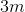above the ground. If no outside forces (except gravity) are acting upon it, what will be its maximum height on the other side?

Greater than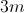Less than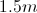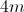Explanation:

If no outside forces act upon the pendulum, it will continue to oscillate back to the original height ofThe proof of this is in the law of conservation of energy. At the top, the pendulum has all potential energy, which is given by the formula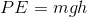. As it swings, the potential energy is converted to kinetic energy until, at the bottommost point, there is only kinetic energy. It then changes direction and begins to rise again. When it rises to the maximum height on the other side, all of its kinetic energy will turn back into potential energy.

Mathematically, the initial and final potential energies are equal.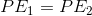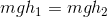Notice the masses and gravity can cancel out on both sides, as neither of these will change. This leaves us with only height.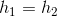### Example Question #2 : Understanding Pendulums

A mass on a string is released and swings freely. Which of the following best explains the energy of the pendulum when the string is perpendicular to the ground?

The mass has maximum potential energy

The mass has equal amounts of kinetic and potential energy

The mass has maximum kinetic energy

The mass has mostly potential energy, but there is some kinetic energy

The mass has mostly kinetic energy, but there is some potential energy

The mass has maximum kinetic energy

Explanation:

Conservation of energy dictates that the total mechanical energy will remain constant. Initially, the mass will not be moving and will be at its highest height. When released, it will begin to travel downward (lose potential energy) and gain velocity (gain kinetic energy). When the mass reaches the bottommost point in the swing, the potential energy will be at a minimum and the kinetic energy will be at a maximum. This point corresponds to the string being perpendicular to the ground.

### Example Question #3 : Understanding Pendulums

A man swings a bucket back and forth at the end of a rope, creating a pendulum. What factor could be used to increase the period of the pendulum?

Use a stronger rope

Move the pendulum to a lower altitude

Remove water from the bucket

Increase the length of the rope

Increase the length of the rope

Explanation:

The equation for the period of a pendulum is: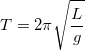Notice that the material of the pendulum and the mass at the end do not enter into the equation at all. The only things that are capable of affecting the period are are the length of the pendulum and the acceleration due to gravity.

While changing the altitude of the pendulum will change the period, it will do it only slightly unless you take it miles above the earth's crust. Furthermore, decreasing the altitude of the pendulum will increase the force due to gravity, which will resulut in a decreased period. The best answer is to increase the length of the rope. This will increase the period of the pendulum.